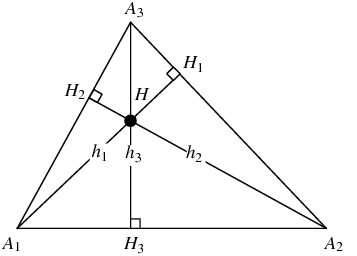# C Program Area Of Triangle | C Programs

C Program to Find the area of a triangle – In this stipulated article, we will brief in on the various methods to calculate the area of a triangle. The ways to calculate the area of a triangle in C programming discussed in this distinct article are as follows:

• Using Standard Method
• Using Function
• Using Pointer
• Using Macros

As we all know, triangles are considered to be a closely bound 2-dimensional with the least number of sides, that is 3. There are many properties of a triangle.

The sum of two sides of a triangle can not be more than that of the third side. The sum of all the angles of a triangle is always 180 degrees.

In order to calculate the area of a triangle, we use this specific formula:

Area of a triangle = 1/2 * Base * Height.

A triangle looks like this:As you can see, calculating the area of the triangle A1A2A3 is extremely easy. The area of the triangle is:

Area of A1A2A3 = 1/2 * A1A2 * A3H3

Thus, the methods discussed in this specific article to find the area of a triangle in C programming are as follows:

## Using Standard Method

1)For calculating the area of a triangle we need height and base of a triangle. The base value will substitute into the variable “base” ,height value in the variable “height”.

2)By substituting base and height in the formula we will calculate the area of a triangle.

output:

### Using Function

1)We are using the function area(float b, float h)to calculate the area of a triangle.

2)area(b,h) calling the function which has float type of arguments.

3)The called function area(float b, float h) will calculate the area and return the value, the return value will be assigned to the variable “a”.

output:

#### Using Pointers

1)We are calling the function by passing references as arguments in the calling function “area(&b,&h,&a)”.

2)The called function void area(float *b, float *h, float *area) calculate the area of a triangle, by retrieving the values at the given addresses and substitute those values in the formula. The calculated value will store into the pointer variable “area”.

output:

##### Using Macros

1)area(b,h) is symbolic name to the expression (b*h)/2.

2)area(b,h) replaced with that expression given at #define.

output:
x

## C Program Inverted Pyramid Star Pattern | 4 Ways – C Programs

C Program to print an inverted pyramid star pattern – In this article, we will ...